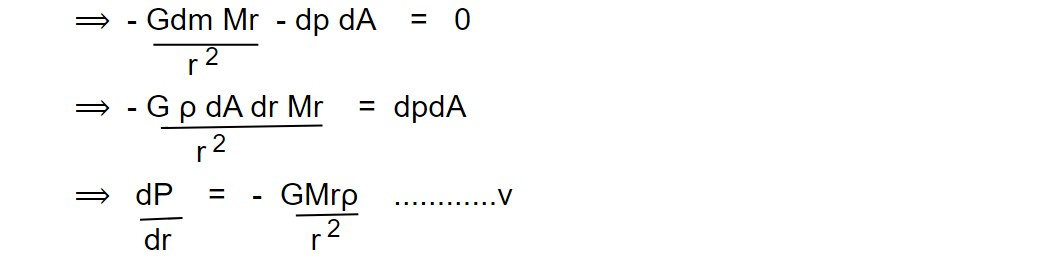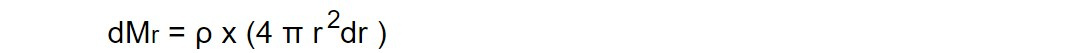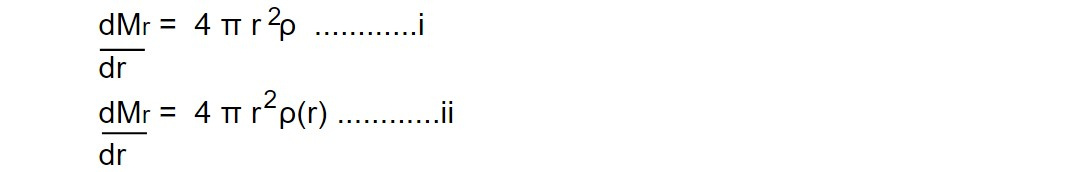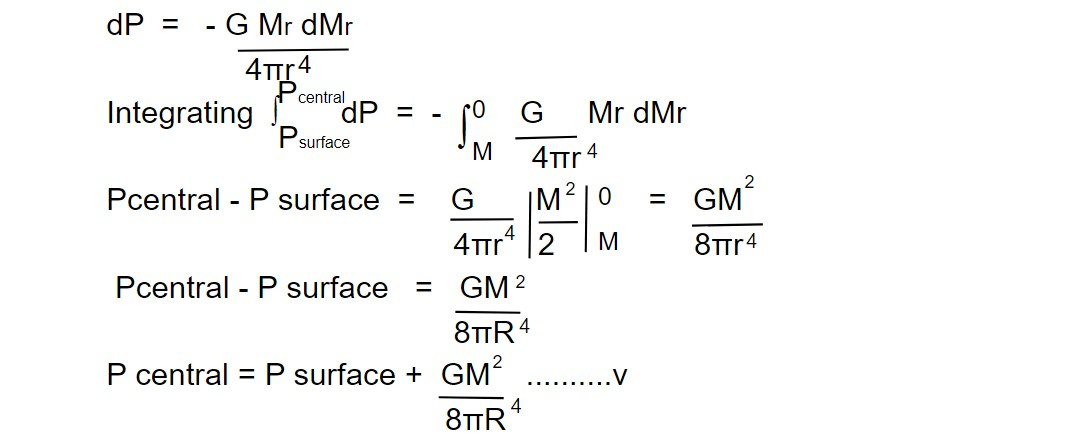# Hydrostatic equilibrium

Hydrostatic equilibrium is an important concept in the study of stars. It refers to the balance between the inward force of gravity and the outward force of pressure that determines the structure of a star. In hydrostatic equilibrium, the pressure at each point in the star is such that it exactly counteracts the force of gravity, preventing the star from collapsing in on itself or expanding outwards.

The maintenance of hydrostatic equilibrium is crucial for the stability and long-term survival of a star. If a star were to lose hydrostatic equilibrium, it could experience significant changes in its structure and evolution, potentially leading to a supernova explosion, the formation of a compact object, or other outcomes.

In summary, hydrostatic equilibrium is a fundamental concept in the study of stars, describing the balance between the forces of gravity and pressure that determines their structure and stability.

Hydrostatic equilibrium occurs when compression due to gravity is balanced by pressure gradient force. The force of gravity pulls the stellar material towards the centre. This gravitational force is resisted by the gas (degenerate and degenerate) and radiation pressure. To be a normal stable star, these forces must be in equilibrium called hydrostatic equilibrium.

Consider a cylindrical volume element at a distance r from the centre. Its volume is

dV = dA x dr .........i

Here we have considered that the difference in the cross section of the base is zero. The mass of this volume element is

dm = ρ dA x dr ........ii

here ρ is assumed to be constant. But in general, density is a function of r, that will be discussed in the Stellar model. If the mass inside r is Mr then the gravitational force (that pulls the stellar material towards the centre) on the volume element will beHere negative sign means that the force is directed towards the centre of the star.

If the pressure at the lower surface of the volume elements is P and the upper surface P+dP, then the net pressure force acting on the volume element i.e

dFp = PdA - (P + dP)dA

= -dpdA .........iv

Since the pressure decreases outwards, dP will be negative and hence dFp will be positive. The equilibrium condition is that the total force acting on the volume element should vanish.

Σ F volume element = 0

=> dFg + dFp = 0The relation deals the first approximation of the balancing condition between the pressure and gravity in the star called hydrostatic equilibrium. In the second approximation, we generally write,Thus pressure gradient increases with r but we know that the pressure decreases outwards.

Mass continuity relation ( Limiting Central pressure )

The mass continuity relation deals the gradient of mass contained within a given radius.

Consider a thin spherical shell of thickness dr at a distance 'r' from the centre. It's mass isHere we have assumed that density is uniform throughout. However, we know that density runs with r, i.e ρ = f(r)Equation i can be used for a particular known density at r. Equation ii can be integrated because it contains density profile [ρ(r)]. According to hydrostatic equations. We haveHere we are not caring density profile,Here, we are not caring density profile,At the surface, P -> 0

For this approximation, we can write,Equation vi. is our required expression for the central limiting pressure.

Previous topic: Pressure exerted by the star

Next topic: Linear Stellar Model

This note is a part of the Physics Repository.